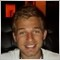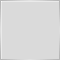# Finding the time of the beginning of the week/ month334

Hey guys,

I just need some help with the logic to this problem I seem to be having, and perhaps it is because I am thinking of this in the incorrect manner?

So, for finding the time of the beginning of the week from any given day within that week, I have simply  used:

int day_of_week=DayOfWeek();
time=Time-Time%(HR2400*day_of_week);

Is this correct?

And then when it comes to finding the beginning of the month I am struggling. I am thinking of doing this but not sure how it would work:

int day_of_month=Day();
int num_days=day_of_month-1;
int bar=iBarShift(NULL,PERIOD_D1,Time-Time%(HR2400*num_days)
datetime time=Time[bar];

But that would include weekends?

Not sure how I would be able to get the integer from the current Day() to the first day of the month so I can use Time[] without including weekends.

Any help is appreciated.Moderator
2308

datetime mthstart=iTime(NULL,PERIOD_MN1,0);
datetime wkstart =iTime(NULL,PERIOD_W1,0);334

honest_knave:
datetime mthstart=iTime(NULL,PERIOD_MN1,0);
datetime wkstart =iTime(NULL,PERIOD_W1,0);

Thanks! I was wondering whether that returned the start of the candle or the current time of that candle which would just be TimeCurrent().

I will use this now, thank you.1647

DeanDeV:

And then when it comes to finding the beginning of the month I am struggling. I am thinking of doing this but not sure how it would work:

Another approach, avoiding the use of timeseries functions, and rounding any date rather than just the current date. Adapted from https://www.mql5.com/en/forum/160249/page3#comment_3818093:

datetime RoundTime(datetime tm, ENUM_TIMEFRAMES tf) {
switch (tf) {
case PERIOD_MN1:
return tm - (tm % 86400) - ((TimeDay(tm) - 1) * 86400);
case PERIOD_W1:
return (((tm - 259200) / 604800) * 604800) + 259200;
default:
return tm - (tm % (PeriodSeconds(tf)));
}
}

For example:

datetime StartOfMonth = RoundTime(TimeCurrent(), PERIOD_MN1);

In other words: for periods below W1, it's just a question of rounding down to the multiple of the number of seconds, such as 3600 (for H1).

This doesn't work with W1 because dates start from 1/1/1970 which was a Thursday. Simple rounding therefore creates a Thu-Wed week. The "259200" in the above code shifts things so that it rounds to a Sun-Sat week. An entirely different approach is required for MN1; there is no simple rounding because months are of different lengths. Therefore, the code rounds to the start of the current day and then subtracts the number of seconds for each prior day in the month so far.Moderator
2308

datetime StartOfMonth(datetime when)
{
MqlDateTime dt;
TimeToStruct(when,dt);
dt.day  = 1;
dt.hour = 0;
dt.min  = 0;
dt.sec  = 0;
return(StructToTime(dt));
}1647

honest_knave:
datetime StartOfMonth(datetime when)
{
MqlDateTime dt;
TimeToStruct(when,dt);
dt.day  = 1;
dt.hour = 0;
dt.min  = 0;
dt.sec  = 0;
return(StructToTime(dt));
}

That, of course, also works.

But, for people concerned with squeezing every last second out of some pointless mass-optimization backtest, bouncing a date in and out of MqlDateTime via TimeToStruct() and then StructToTime() is orders of magnitude slower than what I'm proposing. No significant difference for any scenario outside of backtesting.Moderator
2308

JC:

That, of course, also works.

But, for people concerned with squeezing every last second out of some pointless mass-optimization backtest, bouncing a date in and out of MqlDateTime via TimeToStruct() and then StructToTime() is orders of magnitude slower than what I'm proposing. No significant difference for any scenario outside of backtesting.

True. Many ways to skin the proverbial cat.5

```enum ENUM_CRITERIA_TYPE
{
D1,       // PERIOD_D1
W1,       // PERIOD_W1
MN1,      // PEIROD_MN1
Y1,       // PERIOD_Y1
};

datetime GetCriteriaTime(datetime t, ENUM_CRITERIA_TYPE TYPE)
{
datetime time;
switch(TYPE)
{
case Y1:
time = t - (t % 86400) - ((TimeDayOfYear(t)-1) * 86400);
while(TimeYear(t)!=TimeYear(iTime(Symbol(),0,iBarShift(Symbol(),0,time)))) time=time+Period();
return time;
break;
case MN1:
time=t -(t%86400) -((TimeDay(t)-1)*86400);
while(iBarShift(Symbol(),Period(),time,true)==-1) time=time+Period();
return time;
break;
case W1:
return t - (t % 86400) - (TimeDayOfWeek(t) * 86400);
break;
case D1:
return t - (t % 86400);
break;
default:
return t - (t % (PeriodSeconds(Period())));
}
}```

This function is able to find first "tradable" datetime in each timeframe.

Hopefully it is useful20228

DeanDeV: I was wondering whether that returned the start of the candle or the current time of that candle which would just be TimeCurrent().
```datetime mthstart=iTime(NULL,PERIOD_MN1,0);
datetime wkstart =iTime(NULL,PERIOD_W1,0);```
1. It returns the start of the candle. There is no current time of a candle. The actual start time of a candle (the first tick of a candle) must be remembered (or the first minute found on M1 chart.) The week starts on Sunday - first tick at 5PM. The Month starts on the first - if that is Saturday, first tick is Sunday.